Open in App
Not now

# Node.js MySQL Insert into Table

• Difficulty Level : Hard
• Last Updated : 07 Oct, 2021

NodeJs: An open-source platform for executing javascript code on the server side. Also, a javascript runtime built on Chrome’s V8 JavaScript engine. It can be downloaded from here. Mysql  An open-source Relational Database Management System (RDBMS) that uses Structured Query Language (SQL). It is the most popular language for adding, accessing, and managing content in a database. Here we will use the Mysql as a database for our node application. It can be downloaded from here.

In this article, we are going to learn how to insert rows into an SQL table using Node.js.With the help of SQL INSERT Query.

Initialize Node.js project:

`npm init`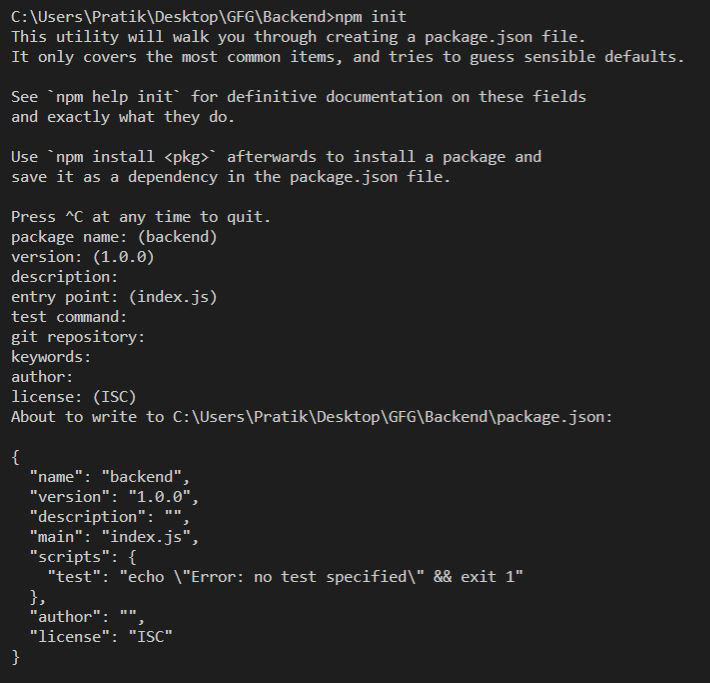Installing Modules:

```npm install express
npm install mysql```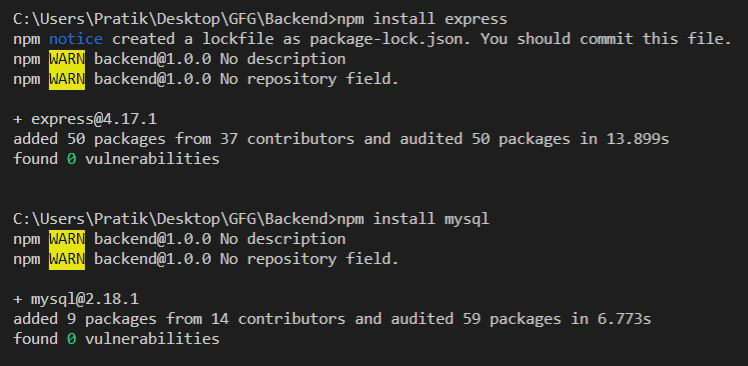File Structure: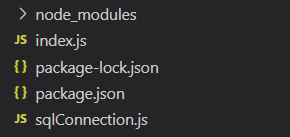MySQL Database Structure:

```gfg_db DATABASE.
gfg_table (id INT AUTO_INCREMENT PRIMARY KEY,

## sqlConnection.js

 `// Importing MySQL module``const mysql = require(``"mysql"``);`` ` `// Creating connection``let db_con = mysql.createConnection({``  ``host: ``"localhost"``,``  ``user: ``"root"``,``  ``password: ``""``,``  ``database: ``"gfg_db"``});`` ` `// Connect to MySQL server``db_con.connect((err) => {``  ``if` `(err) {``    ``console.log(``"Database Connection Failed !!!"``, err);``  ``} ``else` `{``    ``console.log(``"connected to Database"``);``  ``}``});`` ` `module.exports = db_con;`

Anytime we want to make a query we will import the db_con module in that file. This will increase the modularity of our code.

## index.js

 `const express = require(``"express"``);``const database = require(``'./sqlConnection'``);`` ` `const app = express();`` ` `app.listen(5000, () => {``  ``console.log(`Server is up and running on 5000 ...`);``});`` ` `// Use Route Function from below Examples Here...`` ` `app.get(``"/"``, (req, res) => {`` ` `    ``// Call Route Function Here...``});`

Example:

Inserting Single Row: Below is a Route function to insert single row.

## Javascript

 `// Function to insert single row values in``// the database``let singleRowInsert = () => {`` ` `    ``let query = `INSERT INTO gfg_table ``        ``(name, address) VALUES (?, ?);`;`` ` `    ``// Value to be inserted``    ``let userName = ``"Pratik"``;``    ``let userAddress = ``"My Address"``;`` ` `    ``// Creating queries``    ``db_con.query(query, [userName, ``    ``userAddress], (err, rows) => {``        ``if` `(err) ``throw` `err;``        ``console.log(``"Row inserted with id = "``            ``+ rows.insertId);``    ``});``};`

Output: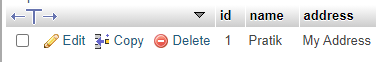Console output:

`Row inserted with id = 1`

Inserting Multiple Rows: Below is a Route function to insert multiple rows.

## Javascript

 `// Function to insert multiple Row in database``let multipleRowInsert = () => {`` ` `    ``// Query to insert multiple rows``    ``let query = `INSERT INTO gfg_table ``        ``(name, address) VALUES ?;`;`` ` `    ``// Values to be inserted``    ``let values = [``        ``[``'Amit'``, ``'Yellow Park'``],``        ``[``'Rishi'``, ``'Park 38'``],``        ``[``'Akash'``, ``'Central st 954'``],``        ``[``'Pratik'``, ``'Road 989'``],``        ``[``'Mangesh'``, ``'Sideway'``]``    ``];`` ` `    ``// Executing the query``    ``db_con.query(query, [values], (err, rows) => {``        ``if` `(err) ``throw` `err;``        ``console.log(``"All Rows Inserted"``);``    ``});``};`

Database Output: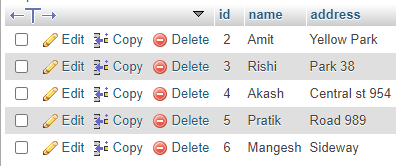Console output:

`All Rows Inserted`

My Personal Notes arrow_drop_up